PHP 一致性hash算法初研究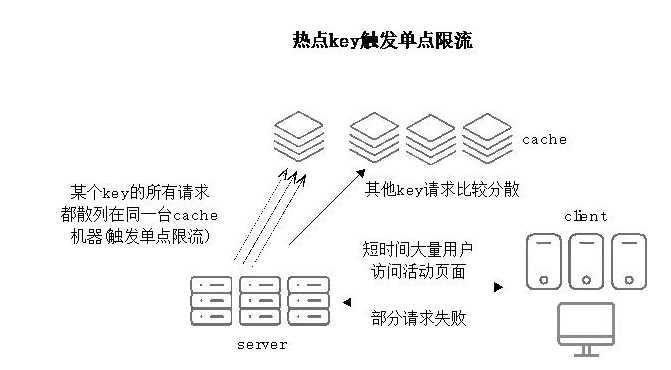1、当所有用户都请求同一个key的时候 如图所示，会导致缓存限流，从而访问db，产生雪崩，最后服务器负载严重，从而使业务垮掉

2、当增加或者删减缓存服务器时，如何最大化的不令数据重新分布。

一致性哈希算法在1997年由麻省理工学院提出的一种分布式哈希（DHT）实现算法，设计目标是为了解决因特网中的热点(Hot spot)问题，初衷和CARP十分类似。一致性哈希修正了CARP使用的简 单哈希算法带来的问题，使得分布式哈希（DHT）可以在P2P环境中真正得到应用。

一致性hash算法提出了在动态变化的Cache环境中，判定哈希算法好坏的四个定义：

1、平衡性(Balance)：平衡性是指哈希的结果能够尽可能分布到所有的缓冲中去，这样可以使得所有的缓冲空间都得到利用。很多哈希算法都能够满足这一条件。

2、单调性(Monotonicity)：单调性是指如果已经有一些内容通过哈希分派到了相应的缓冲中，又有新的缓冲加入到系统中。哈希的结果应能够保证原有已分配的内容可以被映射到原有的或者新的缓冲中去，而不会被映射到旧的缓冲集合中的其他缓冲区。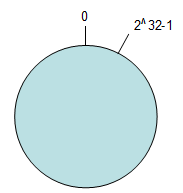Hash(object1) = key1；

Hash(object2) = key2；

Hash(object3) = key3；

Hash(object4) = key4；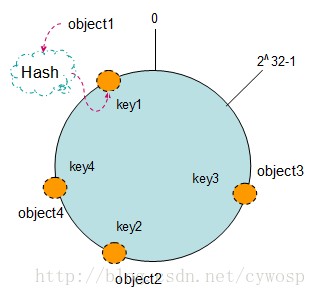Hash(NODE1) = KEY1;

Hash(NODE2) = KEY2;

Hash(NODE3) = KEY3;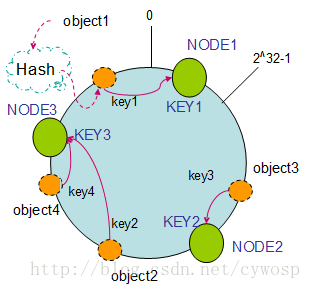1. 节点（机器）的删除

以上面的分布为例，如果NODE2出现故障被删除了，那么按照顺时针迁移的方法，object3将会被迁移到NODE3中，这样仅仅是object3的映射位置发生了变化，其它的对象没有任何的改动。如下图：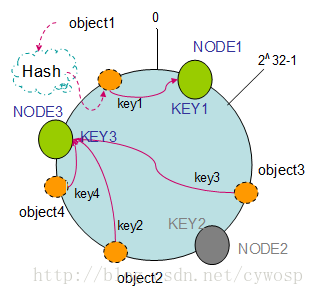2. 节点（机器）的添加

如果往集群中添加一个新的节点NODE4，通过对应的哈希算法得到KEY4，并映射到环中，如下图：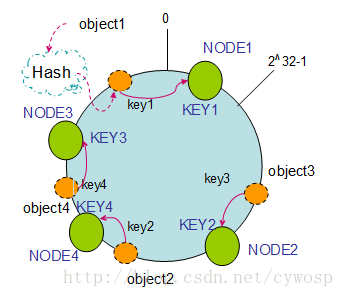通过按顺时针迁移的规则，那么object2被迁移到了NODE4中，其它对象还保持这原有的存储位置。通过对节点的添加和删除的分析，一致性哈希算法在保持了单调性的同时，还是数据的迁移达到了最小，这样的算法对分布式集群来说是非常合适的，避免了大量数据迁移，减小了服务器的的压力。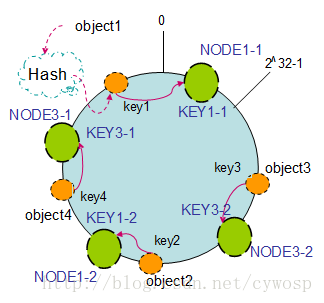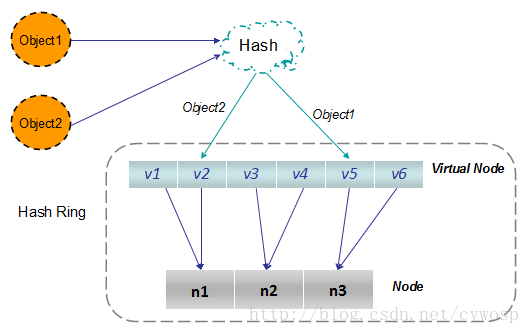hash(i) = hash(i-1) * 33 + str[i]

Time33在效率和随机性两方面上俱佳。对于一个Hash函数，评价其优劣的标准应为随机性，即对任意一组标本，进入Hash表每一个单元（cell）之概率的平均程度，因为这个概率越平均，数据在表中的分布就越平均，表的空间利用率就越高。

PHP

function myHash(\$str) {
// hash(i) = hash(i-1) * 33 + str[i]
\$hash = 5381;
\$s    = md5(\$str); //相比其它版本，进行了md5加密
\$seed = 5;
\$len  = 32;//加密后长度32
for (\$i = 0; \$i < \$len; \$i++) {
// (hash << 5) + hash 相当于 hash * 33
//\$hash = sprintf("%u", \$hash * 33) + ord(\$s{\$i});
//\$hash = (\$hash * 33 + ord(\$s{\$i})) & 0x7FFFFFFF;
\$hash = (\$hash << \$seed) + \$hash + ord(\$s{\$i});
}

return \$hash & 0x7FFFFFFF;
}

5381（001 010 100 000 101），据说hash后的分布更好一些

``````<?php
function myHash(\$str) {
// hash(i) = hash(i-1) * 33 + str[i]
\$hash = 5381;
\$s    = md5(\$str); //相比其它版本，进行了md5加密
\$seed = 5;
\$len  = 32;//加密后长度32
for (\$i = 0; \$i < \$len; \$i++) {
// (hash << 5) + hash 相当于 hash * 33
//\$hash = sprintf("%u", \$hash * 33) + ord(\$s{\$i});
//\$hash = (\$hash * 33 + ord(\$s{\$i})) & 0x7FFFFFFF;
\$hash = (\$hash << \$seed) + \$hash + ord(\$s{\$i});
}

return \$hash & 0x7FFFFFFF;
}

echo "key1: " . myHash("key1") . "<br />";
echo "key2: " . myHash("key2") . "<br />";
echo "key3: " . myHash("key3") . "<br />";
echo "server1: " . myHash("server1") . "<br />";
echo "server2: " . myHash("server2") . "<br />";
echo "server3: " . myHash("server3") . "<br />";

\$data["key1"] = myHash("key1");
\$data["key2"] = myHash("key2");
\$data["key3"] = myHash("key3");
\$data["server1"] = myHash("server1");
\$data["server2"] = myHash("server2");
\$data["server3"] = myHash("server3");
asort(\$data);
var_dump(\$data);

class ConsistentHash {
// server列表
private \$_server_list = array();
private \$_layze_sorted = FALSE;

\$hash = myHash(\$server);
\$this->_layze_sorted = FALSE;

if (!isset(\$this->_server_list[\$hash])) {
\$this->_server_list[\$hash] = \$server;
}
return \$this;
}

public function find(\$key) {
// 排序
if (!\$this->_layze_sorted) {
ksort(\$this->_server_list);
\$this->_layze_sorted = TRUE;
}

\$hash = myHash(\$key);
\$len  = sizeof(\$this->_server_list);
if (\$len == 0) {
return FALSE;
}

\$keys   = array_keys(\$this->_server_list);
\$values = array_values(\$this->_server_list);

// 如果不在区间内，则返回第一个server
if (\$hash <= \$keys || \$hash >= \$keys[\$len - 1]) {
return \$values;;
}

foreach (\$keys as \$key=>\$pos) {
\$next_pos = NULL;
if (isset(\$keys[\$key + 1]))
{
\$next_pos = \$keys[\$key + 1];
}

if (is_null(\$next_pos)) {

return \$values[\$key];
}
// 区间判断
if (\$hash >= \$pos && \$hash <= \$next_pos) {
return \$values[\$key+1];
}
}
}
}

\$consisHash = new ConsistentHash();
echo "<br />";
echo "key1 at " . \$consisHash->find("key1") . "<br />";
echo "key2 at " . \$consisHash->find("key2") . "<br />";
echo "key3 at " . \$consisHash->find("key3") . "<br />";

?>
``````

http://blog.csdn.net/cywosp/article/details/23397179

https://www.toutiao.com/a6492907641960923661/?tt\_from=weixin&utm\_campaign=client\_share&app=news\_article&utm\_source=weixin&iid=17371752795&utm\_medium=toutiao\_ios&wxshare\_count=1blmius
1年前
MySQL:[Err] 1292 - Incorrect datetime value: ‘0000-00-00 00:00:00‘ for column ‘CREATE_TIME‘ at row 1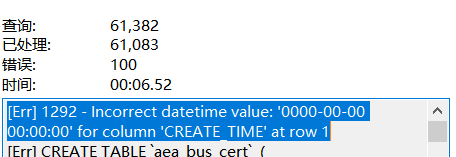Jacquelyn38
2年前
2020年前端实用代码段，为你的工作保驾护航Easter79
1年前
swap空间的增减方法
（1）增大swap空间去激活swap交换区：swapoff v /dev/vg00/lvswap扩展交换lv：lvextend L 10G /dev/vg00/lvswap重新生成swap交换区：mkswap /dev/vg00/lvswap激活新生成的交换区：swapon v /dev/vg00/lvswaphelloworld_34035044
11个月前

​在我们用皕杰报表工具设计填报报表时，如何在新增行里自动增加id呢？能新增整数排序id吗？目前可以在新增行里自动增加id，但只能用uuid函数增加UUID编码，不能新增整数排序id。uuid函数说明：获取一个UUID，可以在填报表中用来创建数据ID语法：uuid()或uuid(sep)参数说明：sep布尔值，生成的uuid中是否包含分隔符''，缺省为Wesley13
1年前
mysql设置时区
mysql设置时区mysql\_query("SETtime\_zone'8:00'")ordie('时区设置失败，请联系管理员！');中国在东8区所以加8方法二：selectcount(user\_id)asdevice,CONVERT\_TZ(FROM\_UNIXTIME(reg\_time),'08:00','0Wesley13
1年前
PHP创建多级树型结构
<!lang:php<?php\$areaarray(array('id'1,'pid'0,'name''中国'),array('id'5,'pid'0,'name''美国'),array('id'2,'pid'1,'name''吉林'),array('id'4,'pid'2,'nWesley13
1年前
00：Java简单了解Stella981
1年前Wesley13
1年前
MySQL部分从库上面因为大量的临时表tmp_table造成慢查询京东云开发者
6个月前Stella981
Lv1

1.7w

32

26